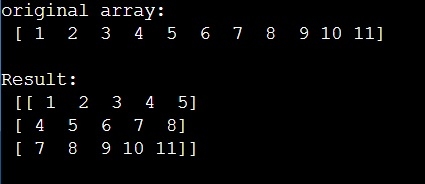# How to take subarrays from NumPy array with given stride/stepsize?

Given a NumPy array, we have to take subarrays from it with given stride/stepsize.
Submitted by Pranit Sharma, on March 14, 2023

## NumPy Array - Taking subarrays with given stride/stepsize

A subarray is part of an array that contains a random set of elements in the original sequence. For example, for an array [1,2,3,4,5], the sub-arrays can be [1,2,3], [2,3,4], etc. Note that [1,3,5] can not be a subarray for this array.

Suppose that we are given a numpy array and we need to create a matrix of sub-sequences from this array of length 5 with stride 3.

Here, length refers to the number of elements in a subarray and stride refers to the number of subarrays we need to create.

To take subarrays from NumPy array with given stride/stepsize, we can use the broadcasting technique by adding a new axis using the None in indexing. Also, we can define the number of rows using the size of the original array, the length of the subarrays, and the strides.

Let us understand with the help of an example,

## Python code to take subarrays from NumPy array with given stride/stepsize

```# Import numpy
import numpy as np

# Creating a numpy array
arr = np.array([ 1,  2,  3,  4,  5,  6,  7,  8,  9, 10, 11])

# Display original array
print("original array:\n",arr,"\n")

# Defining a function
def fun(a, L, S):
rows = ((a.size-L)//S)+1
n = a.strides
return np.lib.stride_tricks.as_strided(a, shape=(rows,L), strides=(S*n,n))

# Getting a subarray matrix
res = fun(arr,5,3)

# Display result
print("Result:\n",res)
```

### OutputLanguages: » C » C++ » C++ STL » Java » Data Structure » C#.Net » Android » Kotlin » SQL
Web Technologies: » PHP » Python » JavaScript » CSS » Ajax » Node.js » Web programming/HTML
Solved programs: » C » C++ » DS » Java » C#
Aptitude que. & ans.: » C » C++ » Java » DBMS
Interview que. & ans.: » C » Embedded C » Java » SEO » HR
CS Subjects: » CS Basics » O.S. » Networks » DBMS » Embedded Systems » Cloud Computing
» Machine learning » CS Organizations » Linux » DOS
More: » Articles » Puzzles » News/Updates